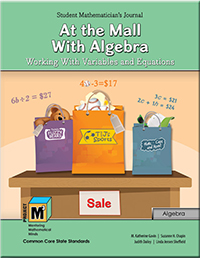# Project M³ Curriculum Units — Level 4-5

## At the Mall With Algebra: Working With Variables and Equations

(get the book choose grades 4-5)Although when we hear the word algebra, we generally think about the high school curriculum, the Common Core State Standards (2010) and the National Council of Teachers of Mathematics (2000) have elevated this content area to one of great importance across the K–12 mathematics curriculum. In the early grades, the emphasis should be on developing algebraic reasoning, and that is what this unit emphasizes.

In many respects, algebra is the generalization of arithmetic, and so we encourage students to approach the study of variables, expressions and equations using their number sense, logical reasoning and problem-solving strategies. We introduce students to these concepts through interesting problem-solving situations. They are intrigued to figure out the mathematics behind number tricks and to solve variable puzzles. In discovering the answers to these problems, students learn about different ways to represent and solve similar types of problems using variables, expressions and equations.

As students represent and analyze mathematical situations using algebraic symbols, they come to understand the basic notions of equality and equivalent expressions. They learn how variables are used to represent change in quantities and also to represent a specific unknown in an equation. The idea that the same variable represents the same quantity in a given equation or set of equations is a fundamental algebraic concept that students will use throughout their mathematical learning. In this unit students’ understanding of these concepts comes out of informal problem-solving in which they use mathematics to make sense of the situations posed, just like real mathematicians.